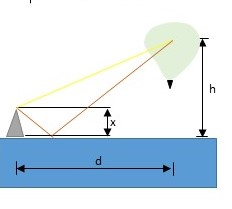# A radar tower sends out a signal of wavelength \lambda. It is x meters tall, and it stands on...

## Question:

A radar tower sends out a signal of wavelength {eq}\lambda. {/eq} It is x meters tall, and it stands on the edge of the ocean. A weather balloon is released from a boat that is a distance d out to sea. The balloon floats up to an altitude h. In this problem, assume that the boat and balloon are so far away from the radar tower that the small-angle approximation holds.

a) Due to interference with reflections off the water, certain wavelengths will be weak when they reach the balloon. What is the maximum wavelength {eq}\lambda {/eq} that will interfere destructively?

b) What is the maximum wavelength {eq}\lambda {/eq} that will interfere constructively?## Interference of Waves:

If two coherent waves of the same frequency interact, they form a pattern of low and high intensity spots in space, called interference pattern. Whenever the path length difference between two waves is such that two waves are in phase, the amplitude of the resulting signal is the sum of two amplitudes and the intensity is at the maximum. Whenever the path length equals the half of the wavelength, the two waves cancel each other and we have a minimum of intensity, corresponding to the destructive interference.

The path length difference between two waves reaching the balloon is given by:

{eq}\Delta = 2 x sin \theta {/eq}

Here

• {eq}\theta = arctan \left ( \dfrac {h}{d} \right ) {/eq} is the angle above horizon the balloon is at as viewed from the bottom of the tower.

In the small angle approximation, the tangent can be considered to be approximately equal to the angle itself. Then we can write:

{eq}\Delta = \dfrac {2xh}{d} {/eq}

a) In the case of the destructive interference, we obtain the following condition:

{eq}\Delta = \left (m + \dfrac {1}{2} \right ) \lambda {/eq}

The maximum wavelength interfering destructively is given by:

{eq}\lambda_{max} = 2\Delta = \boxed {\dfrac {4xh}{d}} {/eq}

b) In the case of constructive interference, the condition for such interference to occur is as follows:

{eq}\Delta = m \lambda {/eq}

The maximum wavelength interfering constructively is given by the following expression:

{eq}\lambda_{max} = \boxed {\dfrac {2xh}{d}} {/eq}